# Secant-squared function

Jump to: navigation, search
This article is about a particular function from a subset of the real numbers to the real numbers. Information about the function, including its domain, range, and key data relating to graphing, differentiation, and integration, is presented in the article.
View a complete list of particular functions on this wiki
For functions involving angles (trigonometric functions, inverse trigonometric functions, etc.) we follow the convention that all angles are measured in radians. Thus, for instance, the angle of$90\,^\circ$ is measured as$\pi/2$.

## Definition

The secant-squared function, denoted$\sec^2$, can be defined in the following equivalent ways:

1. It is the composite of the square function and the secant function (which in turn is the composite of the reciprocal function and the cosine function).
2. It is the composite of the reciprocal function and the cosine-squared function.

Explicitly, it is given as: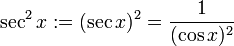$\sec^2 x := (\sec x)^2 = \frac{1}{(\cos x)^2}$

It is defined wherever the secant function is defined, i.e., wherever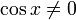$\cos x \ne 0$.

## Key data

Item Value
default domain All real numbers except odd multiples of$\pi/2$.
range$[1,\infty)$, i.e.,$\{ y \mid y \ge 1 \}$
period$\pi$, i.e.,$180\,^\circ$
local minimum values and points of attainment value of 1, attained at all integer multiples of$\pi$.
local maximum values and points of attainment no local maximum value
important symmetries even function (follows from composite of any function with even function is even, and the fact that the secant function is even)
mirror symmetry about lines of the form$x = n\pi/2$,$n$ an integer
first derivative$x \mapsto 2\sec^2x\tan x = 2 (\tan x + \tan^3x)$
second derivative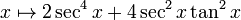$x \mapsto 2\sec^4x + 4\sec^2x \tan^2x$
higher derivatives The$n^{th}$ derivative is expressible as a polynomial in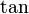$\tan$ of degree$n + 2$.
first antiderivative tangent function, so$x \mapsto \tan x + C$
second antiderivative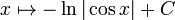$x \mapsto -\ln|\cos x| + C$